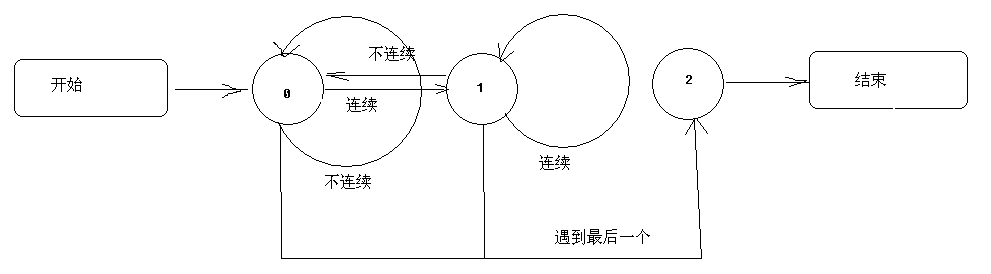Java后台如何判断一串数字是否连续，连续合并数字

``````        输出的结果为 1-3,5,7-10,13
``````
0

2个回答

`````` public class Main {
public static void main(String[] args) {
Integer[] NoNum = {1, 2, 3, 5, 7, 8, 9, 10, 13};
String result = convert(NoNum, 0);
System.out.println(result.substring(0, result.length() - 1));
}

public static String convert(Integer[] ints, int index) {
int end = index;
if (ints.length == index) {//结束条件，遍历完数组
return "";

} else {
for (int i = index; i < ints.length; i++) {
if (i < ints.length - 1) {
if (ints[i] + 1 == ints[i + 1]) {
end = i;
} else {
if (i > index)
end = end + 1;
break;
}
} else {
if (end == ints.length - 2) {
end = ints.length - 1;
break;
}
}
}
if (index == end)//相等说明不连续
return ints[index] + "," + convert(ints, end + 1);
else//连续
return ints[index] + "-" + ints[end] + "," + convert(ints, end + 1);

}

}
}

``````
0JFree_Wolf 回复qq_41877813: 第一个页面的dom传递到第二个页面，第二个页面写值点击确定后，修改之前传过来的dom中的某个区域值，这个值就是第二个页面传过来的。第一个页面再来操作这个值
8 个月之前 回复qq_41877813 回复JFree_Wolf: java前台js怎么从第一个页面获取值传到第二个页面，并不刷新第二个页面
8 个月之前 回复JFree_Wolf 回复qq_41877813: 发了。
8 个月之前 回复qq_41877813 那你也发我吧，谢谢啦
8 个月之前 回复

state 表示状态，0表示开始状态或者前面的是独立的
1表示在连续之中
2表示最后一个``````class Untitled {
public static void main(String[] args) {
int[] NoNum ={1,2,3,5,7,8,9,10,13};
int state = 0;
String result = "";
for (int i = 0; i < NoNum.length; i++)
{
if (i == NoNum.length - 1) state = 2;
if (state == 0)
{
if (NoNum[i + 1] == NoNum[i] + 1)
{
result += Integer.toString(NoNum[i]);
result += "-";
state = 1;
}
else
{
result += Integer.toString(NoNum[i]);
result += ",";
}
}
else if (state == 1)
{
if (NoNum[i + 1] != NoNum[i] + 1)
{
result += Integer.toString(NoNum[i]);
result += ",";
state = 0;
}
}
else
{
result += Integer.toString(NoNum[i]);
}
}
System.out.println(result);
}
}
``````

1-3,5,7-10,13

0weixin_45841714 回复qq_41877813: 这个问题采纳了我的回答，重开一个问题，回答你。
8 个月之前 回复qq_41877813 回复qq_41877813: java前台js怎么从第一个页面获取值传到第二个页面，并不刷新第二个页面
8 个月之前 回复qq_41877813 谢谢大佬
8 个月之前 回复/** * 是否是连续数字 * * @param numOrStr * @return */ public static boolean isOrderNumeric(String numOrStr) { boolean flag = true; for (int i = 0; i < numOrStr.length(); i++) { if (i > 0) {

public static void main(String[] args) { //此种情况已经明确是数字拼接而成,如果可能含有非数字的情况,需要先判断是否全部为数字 String str1= "00001,00002"; String[] split = str1.split(","); for (int i = 0; i < s

1.因为给的是随机数，所以需要剔除重复的数字，然后排序 2.判断是否连续的，就是1 2 3 4 5这样子是否连续 3.因为数组已经是没有重复数据，并且排序好的一组数，所以找到最大的和最小的公式为：(最大-最小)/(数组长度-1)=1 根据这个公式就可以判断是否连续了 还有一种最笨的方法。通过for循环，得到下一个数-当前数=1 ，这种做法就是如果全部连续的，那就需要在for循环中执行数组长
java正则表达式判断一个字符串包含连续数字

php查出连续一组数字中缺少的那个数字

js如何判断一组数字是否连续,得到一个临时数组[[3,4],[13,14,15],,,];
var arrange = function(arr){ var result = [], temp = []; arr.sort(function(source, dest){ return source - dest; }).concat(Infinity).reduce(function(source, dest){ ...

package java程序员面试笔试宝典; import java.util.Arrays; public class 题8_5_17判断相邻数组中数值是否连续 { public static void main(String[] args) { int[] a={0,0,10,0,6}; System.out.println(isContinue_0(a)); } pu...

Co ///         /// 传入的值是否是连续的数字（可重复，但必须是连续的数字）         ///         /// 1,2,3,4,5,6         ///         public static bool GetStrOrder(string str)         {             string[] arry = s

oracle 判断组内数据是否连续，即 等比例连续
--以grid为组，按year大小排序  select t4.*,t4.year-lag(t4.year,1,t4.year) over (partition by t4.grid order by t4.year asc) flag from 表名 t4 lag（）函数取前一次记录。 当前年份t4.year 减去 上一条记录的年份 lag(t4.year,1,t4.y

-
java判断字符串不是相同数字或者相同字母？不能全是连续数字？（密码简易规则校验）...
/** * 不能全是相同的数字或者字母（如：000000、111111、aaaaaa） * @param str str.length()&gt;0 * @return 全部相同返回true */ public static boolean equalStr(String numOrStr){ boolean flag = true; char str = nu...

(Python)判断列表的连续数字范围并分块

from itertools import groupby #获取列表中连续数字序列 lst = [234,55,233,1, 2, 3,4, 5, 6, 7, 8, 11, 12, 13, 19,21344,4646,22,34,55] # 连续数字 index_with_fault = [] fun = lambda x: x-x for k, g in groupby(e...

String str = "【中兴视通】cool170:您的注asdfjaskljhklasdjakl;qweopgm55555fgsdfg,emklemklwe册验证码为9526，请于2分钟内正确输入验证码。"; Pattern pattern = Pattern.compile("(? Matcher matcher = pattern.matcher(str); if(matcher.

java 判断一个数组中的数值是否连续相邻

js/java判断数组中数字连续
js实现判断数组数字连续 function checkValue(a) { for (var i = 0; i &amp;lt; a.length-1; i++) { if(a[i+1]-a[i] != 1){ return false; } } return true; } var a = [1,2,3,4,5,6...

php 判断顺子|判断为三个数字连号|随意3个数字连号判断顺子
\$ss = 123; //你要测试的数字 if(preg_match('/^(0(?=1)|1(?=2)|2(?=3)|3(?=4)|4(?=5)|5(?=6)|6(?=7)|7(?=8)|8(?=9)){2}\d\$/',\$ss)){ echo "是顺子"; }else{ echo "不是顺子"; }
ORACLE_某列连续数判断

java判断循环数组是否连续并获取不连续的项以及重复项
http://blog.csdn.net/tengdazhang770960436/article/details/8183321 package com.hdx.test; import java.util.ArrayList; import java.util.List; public class Lianxu { public static

Oracle数据库如何取连续数据并作分组

oracle产生连续的数字

sql找出连续时间连续日期和连续数字
tips: 1 代码在sqlserver2005实现,其他数据需要经过修改 2 计算连续时间和连续数字是同一类问题,所以合起来一起说,计算连续时间的时候只不过将时间转换成数字而已 3 此方法相对高效 第一步 构造数据 create table #tmptable(id int identity(1,1),rq smalldatetime) insert #tmptable val...
iOS 判断数组中数字是否连续

java获取一维数组中连续数字分成几个连续的数字数组
java获取一维数组中连续数字分成几个连续的数字数组 比如{12,13,14,23,24,34,45,46,47,48,56} 分成【12,13,14】【23,24】【34】【45,46,47，48】【56】等 public static int[][] getContinuousNumber(int[] array) { int[][]arrays=new int[array.l
JAVA 判断是否连续字母或者数字

LeetCode180-MySQL-连续出现的数字